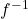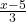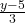Which function represents the inverse of function fbelow? f(x) = 3 x + 5​

Question

Which function represents the inverse of function fbelow?
f(x) = 3 x + 5​

in progress 0
5 months 2021-09-03T06:04:33+00:00 1 Answers 2 views 0(x) =Step-by-step explanation:

let y = f(x) then rearrange making x the subject , that is

y = 3x + 5 ( subtract 5 from both sides )

y – 5 = 3x ( divide both sides by 3 )= x

Change y back into terms of x with x =(x) , then(x) =